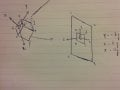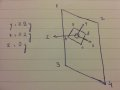# Using IMU Orientation to Detect a Specific Position

Thread Starter

#### Johnny1010

Joined Jul 13, 2014
96
Hi everyone,

I have a rather difficult problem to explain, so I am using some figures to explain what I am trying to do.In the above figure you can see an imaginary wall or a plane 1234 on your right and a cube swinging like a pendulum on the left. I want to detect the moment the cube crosses the plane. An IMU (accelerometer+gyroscope) is placed on the left face (abcd) of the cube and once the cube crosses the plane the face abcd aligns and gets parallel to the 1234 plane. Here, the x & z axis are perpendicular to the gravity thus zero and y is opposite to gravity thus 1. During the swing y & z will be changing their values.

Now I could monitor just either the z-axis or the y axis to see if the plane has been crossed but the IMU could be on any side of the cube like in this figure it is on the front face dcfe. So, in this case the change would occur in x & y.Thus the only option is to monitor y, but apart from moving straight towards the plane the cube can hit the plane at an angle as well i.e. y not being aligned with gravity as was the case in the last 2 figures rather having some angle. Thus making all three axis unusable.Any ideas may be combining the axis values, working with quaternions or something else.

Feel free to ask if the figures or the problem statement is not clear. Thanks in advance.